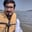Related Tags

oracle
communitycreator

# How to create a table in ORACLEArslan Bajwa

You can create a table in ORACLE database using the CREATE TABLE statement.

## Syntax

The basic syntax is described as follows:

CREATE TABLE schema_name.table_name (
column1 data_type column_constraint,
column2 data_type column_constraint,
...
column_n data_type column_constraint,

table_constraints
);
1. schema_name: represents the name of the schema in which table is to be created. Optional incase of current schema.
2. table_name: represents the name of the table to be created.
3. column1, column2, … column_n: represents the name of the columns to be added in the table.
4. data_type: each column must have a datatype such as NUMBER, FLOAT, or VARCHAR2.
5. column_constraint: each column should be defined as NULL or NOT NULL. Default is NULL incase left blank.
6. table_constraint: Table constraints such as PRIMARY KEY, FOREIGN KEY, and CHECK can be applied to the table if applicable.

## Examples

Below is an example of how to create a simple table in the current schema in ORACLE database:

CREATE TABLE students  (
student_id number(10) NOT NULL,
student_name varchar2(50) NOT NULL
);  

Below is an example of how to create a table with constraints in the current schema:

CREATE TABLE students  (
student_id number(10) NOT NULL,
student_name varchar2(50) NOT NULL,

CONSTRAINT student_key PRIMARY KEY (student_id)
);  

Below is an example of how to create a table with constraints in a different schema:

CREATE TABLE studentsDB.students  (
student_id number(10) NOT NULL,
student_name varchar2(50) NOT NULL,

CONSTRAINT student_key PRIMARY KEY (student_id)
);  

Names can also be written within inverted commas, like “students”.

RELATED TAGS

oracle
communitycreator

CONTRIBUTORArslan Bajwa
RELATED COURSES

View all Courses

Keep Exploring

Learn in-demand tech skills in half the time